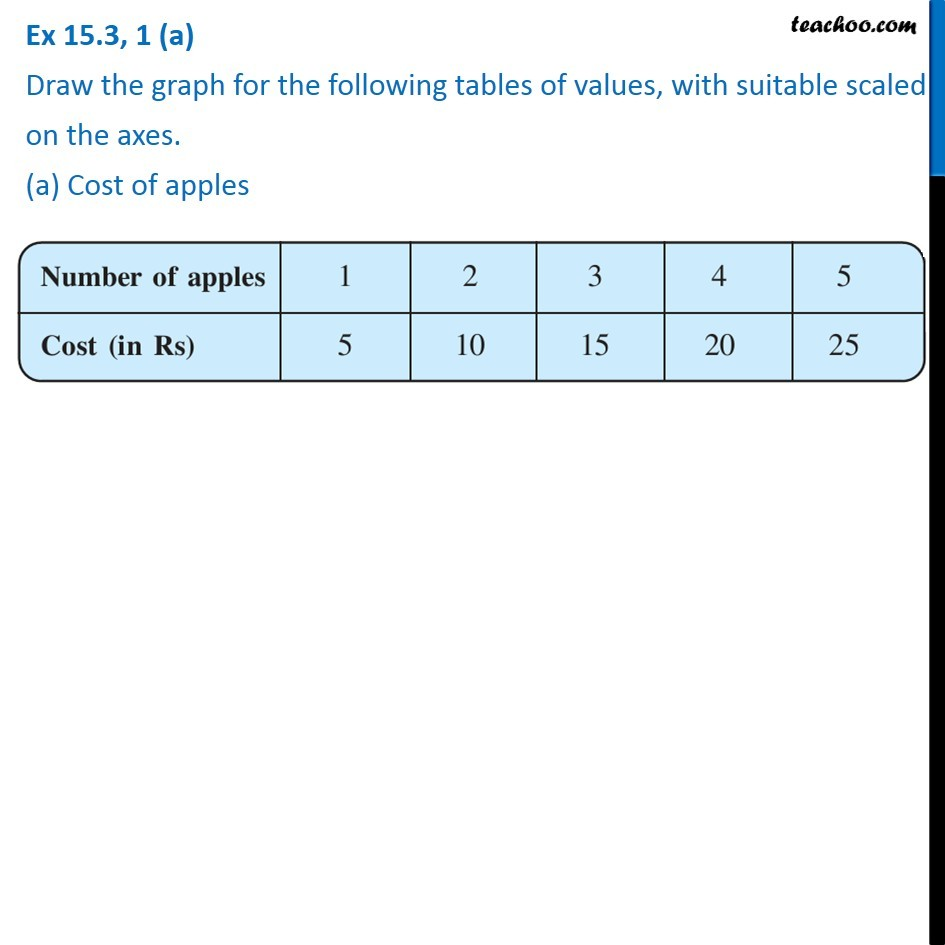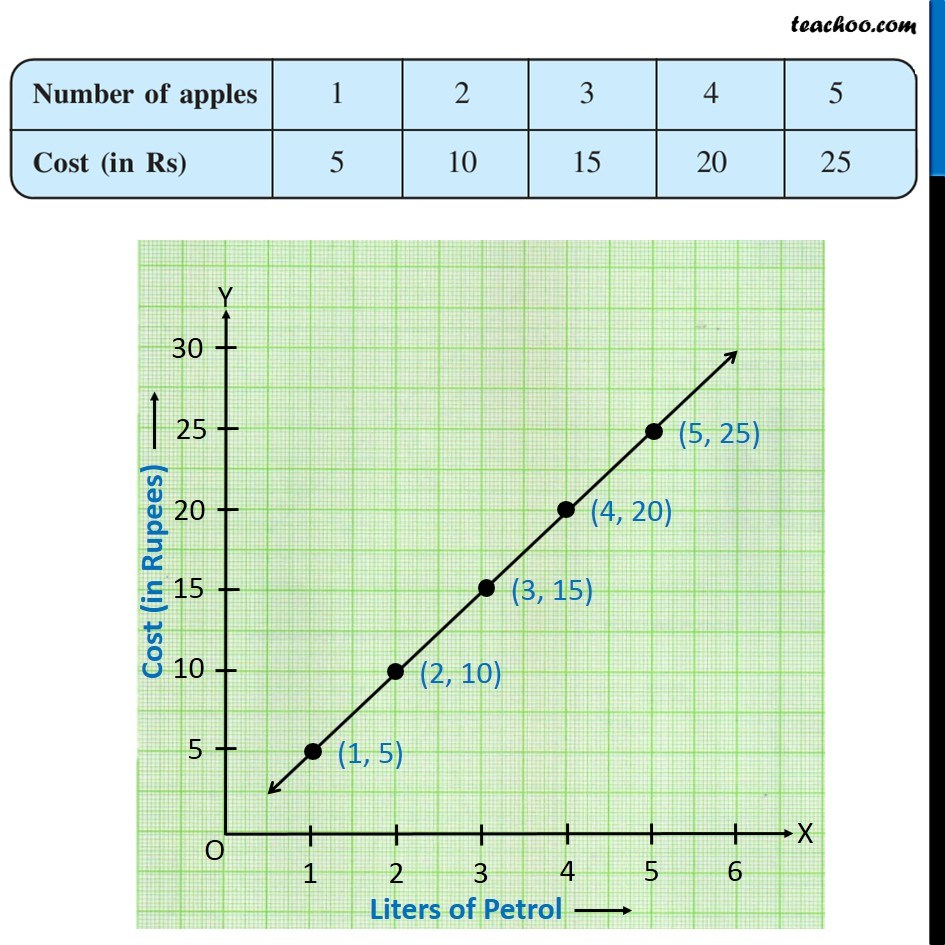Ex 15.3

Chapter 15 Class 8 Introduction to Graphs
Serial order wise### Transcript

Ex 15.3, 1 (a) Draw the graph for the following tables of values, with suitable scaled on the axes. (a) Cost of apples Number of apples | 1 | 2 | 3 | 4 | 5 Cost (in Rs) | 5 | 10 | 15 | 20 | 25 Plotting Number of apples in x-axis, from 0 to 6 (with a gap of 1) and Cost in y-axis, from 0 to 30 (with a gap of 5) The graph is as shown below